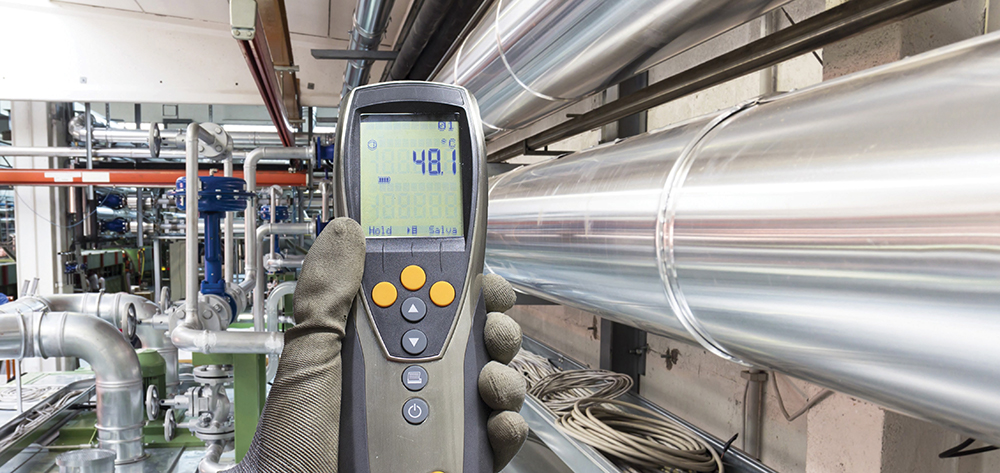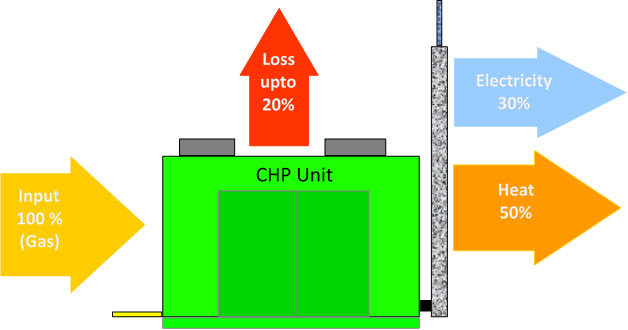# Is Your CHP Unit Making You Savings?

Tags:When you ask a CHP owner why they are operating a CHP unit, they will most often answer ‘because it saves us money’. But does it? How do you know it’s making the savings you expect?

Unless you know how to calculate your CHP savings, you will not be able to answer the question. In this article, we will look at the common methodology to calculate CHP savings.

## What do you need to know?

Before we can calculate CHP savings there are two (2) major requirements, they are:

1. Utility Prices
2. CHP Operational Data

Dealing with each of these in turn:

### Utility Prices

To calculate your CHP savings you will need the following utility data:

• Electricity Day Rate Price (£/kWh)
• Electricity Night Rate Price (£/kWh)
• Gas Price (£/kWh)
• Maintenance Cost (£/kWh)*
• Climate Change Levy – Gas (£/kWh)
• Climate Change Levy – Electricity (£/kWh)

*This is normally a rate charged by the CHP maintainer based on the electricity generated by the CHP unit. This is also known as the CHP electricity price and in most cases does not vary if the CHP operates during the day or the night.

### CHP Operational Data

To calculate your CHP savings you will need the following CHP operational data:

• CHP hours run-in period
• CHP Gas used (kWh) in period
• CHP Electricity generated (kWh) in period
• CHP Heat supplied (kWh) in period

You will also require details of the heat efficiency of the gas-fired backup boiler(s) to the CHP unit.

Once we have the above data for a specific period that we wish to look at, normally monthly, then we can start to calculate the CHP savings.

## CHP Operation

Let’s start by looking at a typical CHP unit and how it operates, this is most easily shown using the following diagram:Simply put, the CHP unit consumes gas to generate electricity and supply heat to the site it is installed at.

For the following calculations we are going to make the following assumptions with regards to the CHP operation and the utility prices:

The CHP unit has the following specification:

 Electrical Output 100 kW Heat Output 150 kW Gas Input 300 kW Daily Run Hours 17 – The CHP unit does not run during the ‘night’ period

Taking the month of January as an example, the CHP unit will operate for 527 hours and generate/consume the following:

 Electricity Generated in period 52,700 kWh Heat Supplied in period 79,050 kWh Gas Consumed in period 158,100 kWh

Finally, before we can start calculating the net savings of the CHP unit in the period, we must know if the CHP unit is registered under the CHPQA Programme.

If a CHP unit is registered under the CHPQA Programme and meets the criteria for good quality CHP, then all the gas used and all the electricity generated is exempt from the climate change levy (CCL). We will for this example assume that the CHP unit is CHPQA registered and is classed as good quality.

For the following example, we will assume the following utility prices at site:

 Electricity Day Rate Price £0.08000/kWh Electricity Night Rate Price £0.04000/kWh Gas Price £0.02000/kWh Maintenance Cost £0.03000/kWh

The Climate Change Levy rates are currently as follows:

 Climate Change Levy – Gas £0.00203/kWh Climate Change Levy – Electricity £0.00583/kWh

We will also assume for the following example that the gas-fired backup boiler heat efficiency is 85%.

We now have all the data we need to calculate the CHP savings. The first step is to calculate the CHP costs.

## CHP Costs

Effectively the total cost to operate the CHP, is the cost of the gas consumed and the cost of the maintenance to operate it; so we have:

 Cost of CHP Gas 158,100 kWh x £0.02/kWh = £3,162.00 Cost of CHP Maintenance 52,700 kWh x £0.03/kWh = £1,581.00 Total CHP Operational Cost = £4,743.00

## CHP Savings

We will now look at the CHP savings. These can be separated into two (2) parts; the electricity savings and the heat savings:

### The Electricity Savings

If there was no CHP unit operating on site, then the electricity generated by the CHP unit would have to be imported from the grid and would include the cost of CCL. So the cost of importing the equivalent electricity from the grid would be:

 Electricity Day Rate Price £0.08000/kWh Climate Change Levy – Electricity £0.00583/kWh Total Electricity Price (Day) £0.08583/kWh

Therefore the total cost to import the equivalent electricity from the grid would be:

 Total Cost Equivalent Grid 52,700 kWh x £0.08583/kWh = £4,523.24

## The Heat Savings

We must also take into account the heat supplied by the CHP unit; for if this was not available then, this heat would have to be supplied the site boiler(s), which would use gas to generate it.

Assuming that the boiler has a heat efficiency of 85%, then the quantity of gas used to generate the equivalent heat would be:

 Equivalent Boiler Gas 79,050 kWh / 0.85 = 93,000 kWh

As the gas used in the boiler is not subject to a CCL exemption, then the CCL rate for gas needs to be included in the price of the gas. So the price of the boiler gas would be:

 Gas Price £0.02000/kWh Climate Change Levy – Gas £0.00203/kWh Total Electricity Price (Day) £0.02203/kWh

Therefore the total cost of the boiler gas used to replace the equivalent heat from the CHP would be:

 Total Boiler Gas Cost 93,000 kWh x £0.02203/kWh = £2,048.79

So this is an effective saving, as this gas does not have to be purchased to generate the heat that is being supplied by the CHP unit.

Therefore the total savings by operating the CHP unit are as follows:

From this we must deduct the Total CHP Operational Cost:

 Total Heat Savings £2,048.79 Total Electricity Saving £4,523.24 Total Energy Cost Saving £6,572.03

 Total CHP Operational Cost £4,743.00 This gives us then a total net CHP Saving of £1,829.03

The above example is based on the optimum performance of the CHP, to calculate your actual CHP savings, then metered values for CHP electricity generated, heat supplied and gas consumed must be used.

2EA Consulting Limited offer a Premium Management Package for clients who operate CHP units that calculates the actual monthly savings your CHP unit is making for you. For more information on this service or provision of a sample report, please see our website and contact us at the address shown.